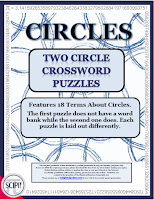### Does A Circle Have Sides?

Believe it or not, this was a question asked by a primary teacher.  I guess I shouldn't be surprised, but in retrospect, I was stunned. Therefore, I decided this topic would make a great blog post.

The answer is not as easy as it may seem. A circle could have one curved side depending on the definition of "side!"  It could have two sides - inside and outside; however this is mathematically irrelevant. Could a circle have infinite sides? Yes, if each side were very tiny. Finally, a circle could have no sides if a side is defined as a straight line. So which definition should a teacher use?

By definition a circle is a perfectly round 2-dimensional shape that has all of its points the same distance from the center. If asked then how many sides does it have, the question itself simply does not apply if "sides" has the same meaning as in a rectangle or square.I believe the word "side" should be restricted to polygons (two dimensional shapes). A good but straight forward definition of a polygon is a many sided shape.  A side is formed when two lines meet at a polygon vertex. Using this definition then allows us to say:

1) A circle is not a polygon.

2) A circle has no sides.

One way a primary teacher can help students learn some of the correct terminology of a circle is to use concrete ways.  For instance,  the perimeter of a circle is called the circumference.  It is the line that forms the outside edge of a circle or any closed curve. If you have a circle rug in your classroom, ask the students is to come and sit on the circumference of the circle. If you use this often, they will know, but better yet understand circumference.

For older students, you might want to try drawing a circle by putting a pin in a board. Then put a loop of string around the pin, and insert a pencil into the loop. Keeping the string stretched, the students can draw a circle!

And just because I knew you wanted to know, when we divide the circumference by the diameter we get 3.141592654... which is the number π (Pi)!  How cool is that?\$2.50
If you are studying circles in your classroom, you might like this resource. It is a set of two circle crossword puzzles that feature 18 terms associated with circles. The words showcased in both puzzles are arc, area, chord, circle, circumference, degrees, diameter, equidistant, perimeter, pi, radii, radius, secant, semicircle, tangent and two. The purpose of these puzzles is to have students practice, review, recognize and use correct geometric vocabulary in a fun, non-threatening way.